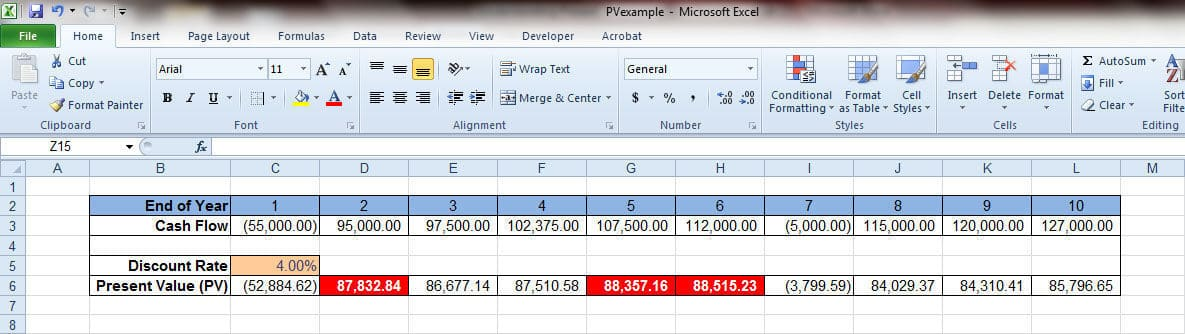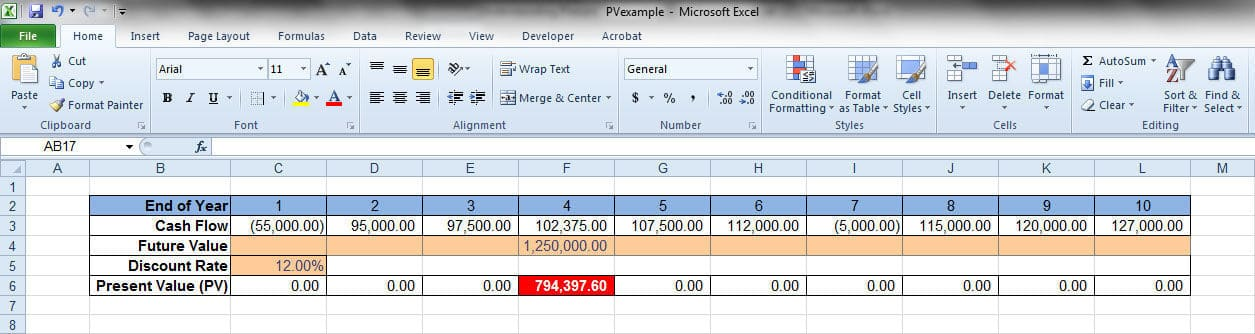# Calculating Present Value with Ease Using ExcelExpertise:
15 Articles WrittenWhat would you do if someone was willing to contribute \$200,000 to help you invest in a multifamily property?  Your first thought would be; what’s the catch?  They tell you, you must wait seven years to receive the money or you may have the money immediately.  You must decide on waiting seven years or receive \$200,000 now?  The answer should be pretty straight-forward, you want the money now.  You can invest that \$200,000 now.  Seven years in the future your investment strategy may have changed.  The \$200,000 may or may not be as important. You know what you can do with the money now.  You cannot predict, with certainty, what you will need seven years into the future.### Want more articles like this?

Create an account today to get BiggerPocket's best blog articles delivered to your inboxThe previous paragraph introduces you to some real estate investing concepts; time value of money, present value and future value. Money that is available to you today for investing is more valuable than money in the future.

What would happen if you were forced to wait the seven years for the \$200,000? Seven years from now, can you predict the economic climate, the depreciation rate or cash flow conditions for multifamily properties? No one can, but if you have a crystal ball that can accurately make those kinds of predictions, please let us all know! Since we cannot predict, seven years into the future, maybe we should just sit and wait for the years to pass by. Some people would take that approach, not real estate investors.

## Predicting the Future

Investors know that they cannot predict the future; investors will still make plans into the future.  Investors look at the value of that \$200,000 that they will receive in the future in today’s present day dollars.  This is where the idea of “time value of money” comes from.  To purchase multifamily properties, the value of the money is affected by when the money is available or the “time”.

Why would an investor care about determining the present value of future investment dollars?  When investing in multifamily properties, the question to be answered is; will the property cash flow?  Cash flow is money in the future.  The cash flow from the property seven years from now is not as valuable as the cash flow you receive today.  Today’s cash flow can be used to purchase more rental properties now.  Today’s cash flow can be used for capital improvements that can lead to raising rents.  Raised rents could mean increased cash flow.

An investor will ask; what is the present value of future cash flows?  This answer will help determine the value of the property in today’s dollars.  With answering this question, how would you know if the price of a property was justified?  How would you know the right time to sell or exchange the property?  In order to answer these questions, you need a way of converting future values into today’s value.

The factor that will convert future cash flow dollars into today’s dollar values is a discount rate. A discount rate will discount the future values back to present values.  An investor would need to determine what discount rate to use.  The best rate would be one that is comparable to the rate of return you would expect from other investments with similar risk factors.  If you did not invest in a rental property, what other investment could you invest in?  This other investment would have a certain rate of return.  That rate of return would be your discount rate to use for future cash flows of the rental property.

## Determining Excel Present Value

To get the present value of future cash flows, you need a formula.  The formula is:

PV = FV/(1 + r)^n

PV is the Present Value, FV is the Future Value, the rate per period is r and the number of periods is n.  That is an intimidating formula that Excel can handle with ease.  Thank goodness, Excel has a Present Value (PV) function where you can enter the numbers and the value is automatically calculated.

Using Excel, we will test the PV() function on a multifamily property covering 10 years of cash flow values.   At the end of each year Excel will calculate the Present Value based upon a Discount Rate of our choosing and sample cash flow values.  The picture below shows a portion of a worksheet that will display the Present Value results.With a fixed set of cash flow values for 10 years, all we need to do is enter a Discount Rate.  The Present Values for each year would be calculated along with the largest three PV values highlighted with a red background.  That was a very straightforward example of using Excel to calculate Present Values.

Present Values can be used in other ways.  For a simple example, assume that we want the Present Value of a single cash flow that you would receive at the end of four years.  Consider a multifamily property that could sell for \$1,250,000 at the end of four years.  The desired rate of return of this property will be 12.0% per year.  What would be the appropriate sales price in today’s dollars in order to have a 12.0% rate of return?## Future Value

The worksheet has an extra row, Future Value.  In this example, the Future Value that we want for the property is \$1,250,000 in the fourth year.  To get the sales price in today’s dollars, the Discount Rate has been changed to 12%.   Time to have Excel solve for the Present Value, in this case it is \$794,397.60.  With a worksheet like the one above, you could enter various Future Values and Excel would calculate the sales price each year based upon your entered Discount Rate.

The Present Value formula in cell F5 is; =-PV(C5,F2,,F4).  Cell C5 is the 12% Discount Rate, cell F2 is the End of Year period and cell F4 is the Future Value of \$1,250,000.  Also notice the PV function is preceded by a negative.  Present Value is negative to denote that it is money that is going out versus Future Value that is money coming in.  The previous example can be stated this way; you purchase the apartment for \$794,398 and after four years you sell it for \$1,250,000 you will earn a rate of return of 12.0% in that year.

It is to your advantage as a real estate investor to understand and use the time value of money, Present Value and other key financial returns.  Having a solid understanding of Excel would allow you to go beyond these one function examples.  Real estate investing can involve many layers of analyses based upon various investing strategies.  Producing one or two quick calculations is only the beginning.

Photo: aussiegalljoe
Excellent Everyone wants too cap rate, but present value is more important.Loretta